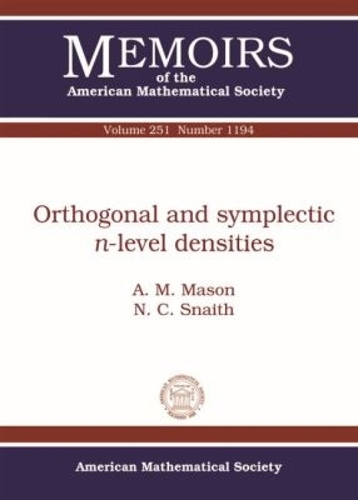•# Orthogonal and Symplectic \$n\$-level Densities - Memoirs of the American Mathematical Society (Paperback)

(author), (author)
£72.50
Paperback 93 Pages / Published: 30/03/2018
• In stock
• Free UK delivery

UK delivery within 2 weeks

In this paper the authors apply to the zeros of families of \$L\$-functions with orthogonal or symplectic symmetry the method that Conrey and Snaith (Correlations of eigenvalues and Riemann zeros, 2008) used to calculate the \$n\$-correlation of the zeros of the Riemann zeta function. This method uses the Ratios Conjectures (Conrey, Farmer, and Zimbauer, 2008) for averages of ratios of zeta or \$L\$-functions. Katz and Sarnak (Zeroes of zeta functions and symmetry, 1999) conjecture that the zero statistics of families of \$L\$-functions have an underlying symmetry relating to one of the classical compact groups \$U(N)\$, \$O(N)\$ and \$USp(2N)\$.

Here the authors complete the work already done with \$U(N)\$ (Conrey and Snaith, Correlations of eigenvalues and Riemann zeros, 2008) to show how new methods for calculating the \$n\$-level densities of eigenangles of random orthogonal or symplectic matrices can be used to create explicit conjectures for the \$n\$-level densities of zeros of \$L\$-functions with orthogonal or symplectic symmetry, including all the lower order terms. They show how the method used here results in formulae that are easily modified when the test function used has a restricted range of support, and this will facilitate comparison with rigorous number theoretic \$n\$-level density results.

Publisher: American Mathematical Society
ISBN: 9781470426859
Number of pages: 93
Dimensions: 254 x 178 mm